• 基于opencv-KNN最邻近算法实现手写数字识别，使用Qt做UI实现手写板，可以实时测试，资源包含源代码和可执行程序（release文件夹下的exe文件可以直接运行）
• 机器学习入门KNN算法实现的手写数字识别 基本上照搬了http://lib.csdn.net/article/opencv/30167的代码，只是改了一点bug和增加了一点功能 输入就是直接在一个512*512大小的白色画布上画黑线，然后转化为01矩阵，用...
• 机器学习实战 KNN 数字识别testDigits 14 16:35:41 CST 2018-数据集数据集
•F#
• 机器学习实战 KNN 数字识别 trainingDigit 14 16:36:51 CST 2018-数据集数据集
• #knn介绍 更多参考百度介绍 算法思想：给定一个带标检的训练数据集（就是带分类结果的样本），对于一个新的输入实例，我们在训练数据集中以某种距离度量方式找出与该输入实例距离最近邻的k个实例。找出这k...

#knn介绍 更多参考百度介绍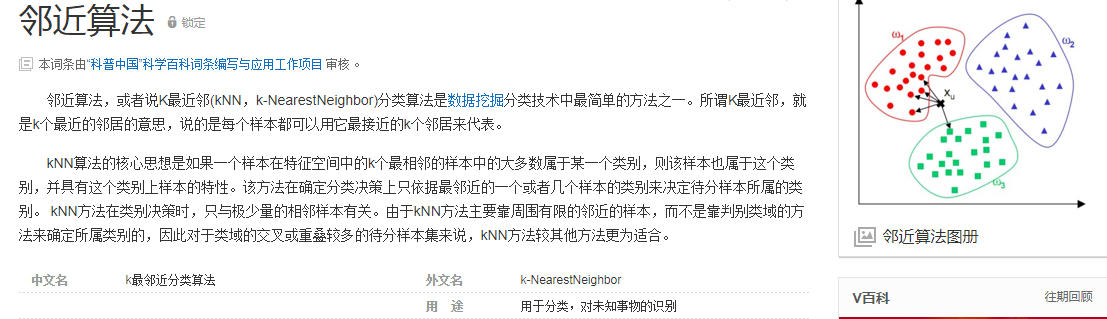算法思想：给定一个带标检的训练数据集（就是带分类结果的样本），对于一个新的输入实例，我们在训练数据集中以某种距离度量方式找出与该输入实例距离最近邻的k个实例。找出这k个实例（这也是knn中k的含义）中类别出现最多的那个类别，最后我们就将该新的输入实例划分为此类别

import numpy as np
# bmp 图片后缀
import matplotlib.pyplot as plt
from sklearn.neighbors import KNeighborsClassifier

img_path = './data/3/3_100.bmp'
arr_img.shape  #维度

#特征必须是二维
feature = [] #特征
target = [] #目标数据
for i in range(10):
for j in range(1,501):
#img_path = './data/'+str(i)+'/'+str(i)+'_'+str(j)+'.bmp'
#将图片数据读取到了numpy
feature.append(img_arr)
target.append(i)
#将列表转numpy
feature = np.array(feature)
feature.shape  #发现feature是三维，必须变形成二维的才可以作为特征数据
#获取了符合要求的特征数据（二维）
feature = feature.reshape((5000,784))
target = target
#将样本集拆分成训练数据和测试数据
np.random.seed(6)
np.random.shuffle(feature)
np.random.seed(6)
np.random.shuffle(target)
#训练数据
x_train = feature[0:4950]
y_train = target[0:4950]
#测试数据
x_test = feature[4950:]
y_test = target[4950:]

x_train.shape  #训练形状
knn = KNeighborsClassifier(n_neighbors=15)
knn.fit(x_train,y_train)  #试数据
knn.score(x_test,y_test)  #评分
print('模型分类结果:',knn.predict(x_test))
print('真实分类:',y_test)

#下面测试一张新的照片

#获取外部的一张数字图片，让模型进行分类
digist_img_arr.shape
plt.imshow(digist_img_arr)
five_img_arr = digist_img_arr[95:150,90:125]  #图片切割   行/列
plt.imshow(five_img_arr)
#检查切分出图片（即将被模型进行分类的图片）的形状
five_img_arr.shape   #(55, 35, 3)

#将图片的第三个维度删除（降维）
five_img_arr = five_img_arr.mean(axis=2) #任意的聚合方法都能降维  0-x 1-y 2-z
five_img_arr.shape
#对不满足像素要求的图片进行等比例压缩
import scipy.ndimage as ndimage
five_img_arr = ndimage.zoom(five_img_arr,zoom=(28/55,28/35))  #图片压缩
five_img_arr.shape #(28, 28)
five_img_arr = five_img_arr.reshape((1,784))  #1行784列
five_img_arr.shape  #(1,784)
knn.predict(five_img_arr)  #X  未知分类的数据

#模型保存和运用

#保存模型
from sklearn.externals import joblib
joblib.dump(knn,'./knn.m')  #写入模型 value, filename, compress=0, protocol=None, cache_size=None
print(kknn)
#KNeighborsClassifier(algorithm='auto', leaf_size=30, metric='minkowski',
#metric_params=None, n_jobs=1, n_neighbors=15, p=2,
#weights='uniform')
kknn.predict(five_img_arr) #测试模型 结果5

转载于:https://www.cnblogs.com/zhangchen-sx/p/11133868.html
展开全文• knn数字识别
knn数字识别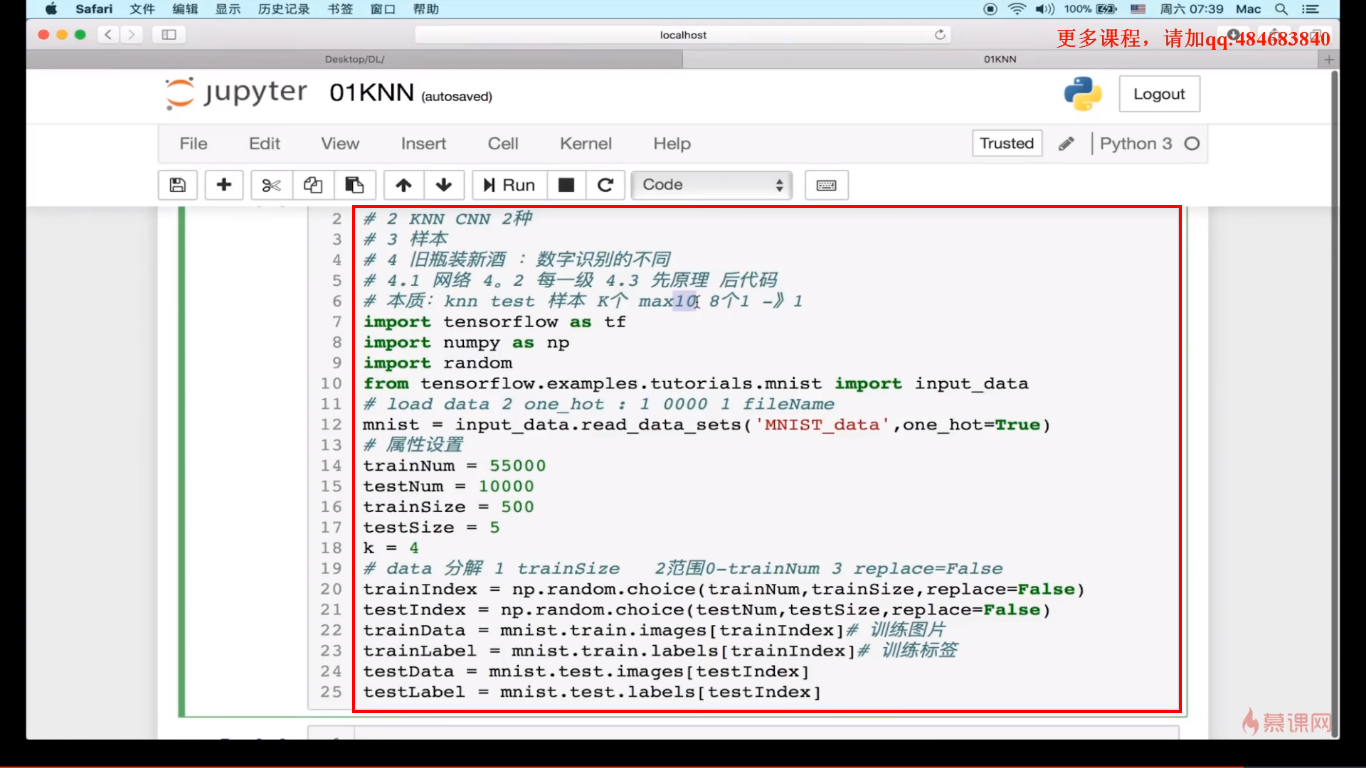展开全文• knn算法实现的数字识别包括 训练集 和 测试集 以及代码
• KNN即K个最近邻，网上有很多关于KNN的文章。我大概总结下核心：假设有A图片，让A与训练样本依次计算相似度（可用欧式距离），挑选出K个与A图片相似度最大的图片，这K个图片中，哪种类型最多那么定义A...KNN数字识...
KNN即K个最近邻，网上有很多关于KNN的文章。我大概总结下核心：假设有A图片，让A与训练样本依次计算相似度（可用欧式距离），挑选出K个与A图片相似度最大的图片，这K个图片中，哪种类型最多那么定义A图片也属于该类型。
首先，需要有数字的训练样本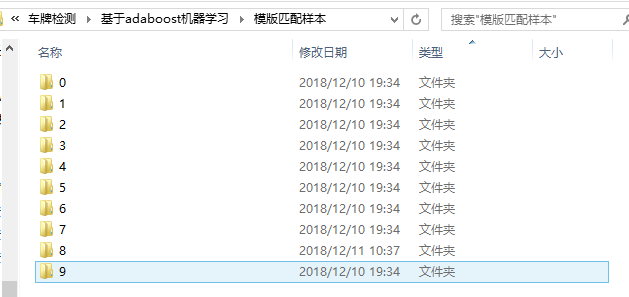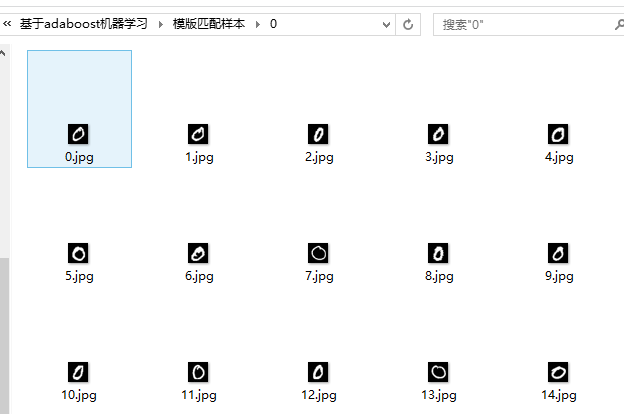KNN的数字识别代码与基于SVM的数字识别大体一致
https://blog.csdn.net/weixin_41721222/article/details/84953788
核心思路：
1：获取一张训练图片后会将图片特征写入到容器中，紧接着会将标签写入另一个容器中，这样就保证了特征和标签是一一对应的关系。
2：特征可用LBP,HOG等提取，但是我们这里主要说KNN训练过程，所以用最简单的方法，即把训练图片的全部像素序列成一行像素作为特征，用reshape（1，1）。
3：图片特征数据得转换成CV_32FC1的数据格式。
4：所有训练样本与测试样本的尺寸都应该一样（这里我都选择20*20）。
下面代码是opencv3和C++
可以根据自己需要修改训练样本类别，数目，尺寸。oss的训练样本路径，src的检测图片路径。

/// 字符识别——基于模版匹配.cpp: 定义控制台应用程序的入口点。
//

#include "stdafx.h"
#include<opencv2/opencv.hpp>
#include<iostream>

using namespace std;
using namespace cv;
using namespace ml;
ostringstream oss;
int num = -1;
Mat dealimage;
Mat src;
int k = 0;
Mat yangben_gray;
Mat yangben_thresh;

int main()
{

===============================读取训练数据===============================
const int classsum = 10;//图片共有10类
const int imagesSum =500;//每类有500张图片
const int imageRows = 20;//图片尺寸
const int imageCols = 20;
//训练数据，每一行一个训练图片
Mat trainingData;
//训练样本标签
Mat labels;
//最终的训练样本标签
Mat clas;
//最终的训练数据
Mat traindata;
//从指定文件夹下提取图片//
for (int p = 0; p < classsum; p++)
{
num += 1;//num从0到9
int label = num;
oss << num << "/*.jpg";//图片名字后缀，oss可以结合数字与字符串
string pattern = oss.str();//oss.str()输出oss字符串，并且赋给pattern
oss.str("");//每次循环后把oss字符串清空
vector<Mat> input_images;
vector<String> input_images_name;
glob(pattern, input_images_name, false);
//为false时，仅仅遍历指定文件夹内符合模式的文件，当为true时，会同时遍历指定文件夹的子文件夹
//此时input_images_name存放符合条件的图片地址
int all_num = input_images_name.size();//文件下总共有几个图片
//cout << num << ":总共有" << all_num << "个图片待测试" << endl;

for (int i = 0; i < imagesSum; i++)
{
threshold(yangben_gray, yangben_thresh, 0, 255, THRESH_OTSU);
input_images.push_back(yangben_thresh);
//循环读取每张图片并且依次放在vector<Mat> input_images内
dealimage = input_images[i];

//注意：我们简单粗暴将整个图的所有像素作为了特征，因为我们关注更多的是整个的训练过程
//，所以选择了最简单的方式完成特征提取工作，除此中外，
//特征提取的方式有很多，比如LBP，HOG等等
//我们利用reshape()函数完成特征提取,
//reshape(1, 1)的结果就是原图像对应的矩阵将被拉伸成一个一行的向量，作为特征向量。
dealimage = dealimage.reshape(1, 1);//图片序列化
trainingData.push_back(dealimage);//序列化后的图片依次存入
labels.push_back(label);//把每个图片对应的标签依次存入
}
}

//图片数据和标签转变下
Mat(trainingData).copyTo(traindata);//复制
traindata.convertTo(traindata, CV_32FC1);//更改图片数据的类型，必要，不然会出错
Mat(labels).copyTo(clas);//复制

===============================创建KNN模型===============================
Ptr<KNearest>knn = KNearest::create();
knn->setDefaultK(10);//k个最近领
knn->setIsClassifier(true);//true为分类，false为回归
//训练数据和标签的结合
Ptr<TrainData>trainData = TrainData::create(traindata, ROW_SAMPLE, clas);
//训练
knn->train(trainData);

//model->save("E:/image/KNearestModel.xml");

===============================预测部分===============================
//预测分类
cvtColor(src, src, COLOR_BGR2GRAY);
threshold(src, src, 0, 255, CV_THRESH_OTSU);
imshow("原图像", src);
Mat input;
src = src.reshape(1, 1);//输入图片序列化
input.push_back(src);
input.convertTo(input, CV_32FC1);//更改图片数据的类型，必要，不然会出错

float r = knn->predict(input);   //对所有行进行预测
cout << r << endl;
waitKey(0);
return 0;
}



结果：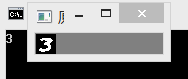展开全文计算机视觉 机器视觉 图像处理
• 主要为大家详细介绍了python使用KNN算法识别手写数字，具有一定的参考价值，感兴趣的小伙伴们可以参考一下
• ## knn算法之数字识别

千次阅读 2019-05-28 21:32:49
目录

（一）训练集文件列表
（二）拿文件，读原数据
（三）数据处理
（四）将上一步的一行1024个0,1赋值给最终的数组（knn计算的数组）
（五）将最终数组进行保存为训练集的训练结果（分类器）
（六）训练集完成（分类器）
（七）测试集制作
（八）进行测试（knn算法）

（一）训练集文件列表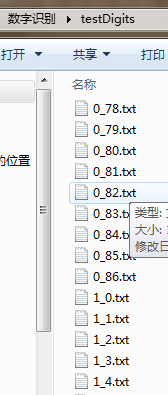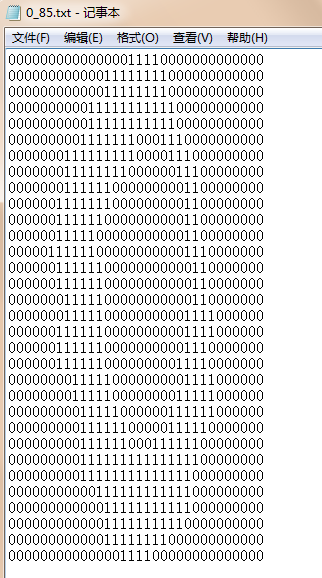32行32列
（二）拿文件，读原数据
这一步的目标是：读取每一个txt，得到原数据
（1）读取小提示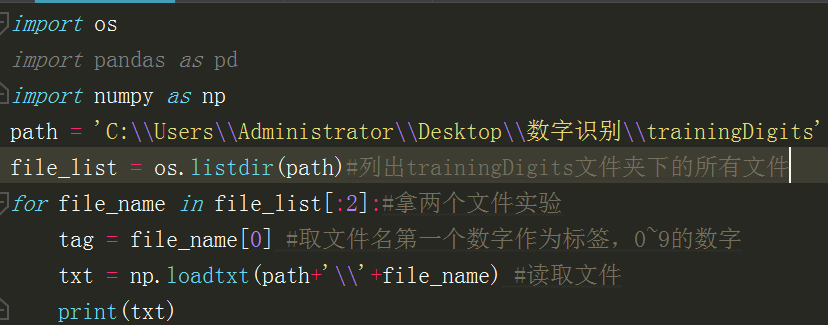输出：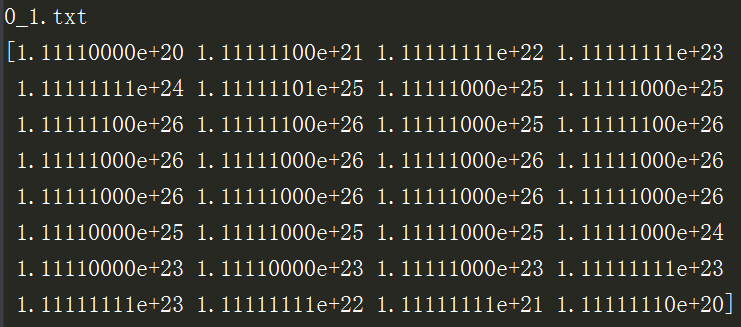默认读出的是科学计数法的数字，我们要得到原来的数据，则在加载文件时加上dtype为str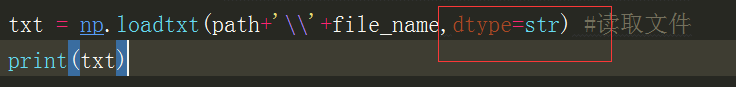结果（原txt文件里每行数据时隔以为列表）：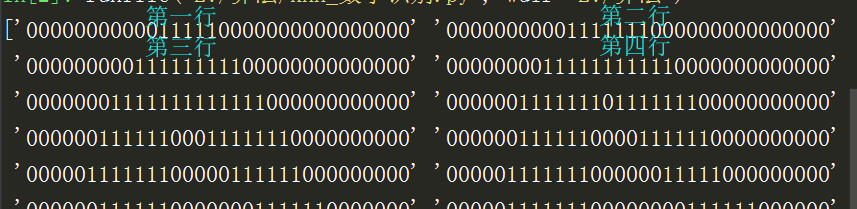（三）数据处理
这一步的目标是：每一个txt的数据（即上图的一维数组）处理为一行1024，即32x32个数字,并且转为int方便knn计算
（1）将上图变成二维数组再展平就是一行了
问题：1、这里一串字符串要变成二维数组的一行，怎么转？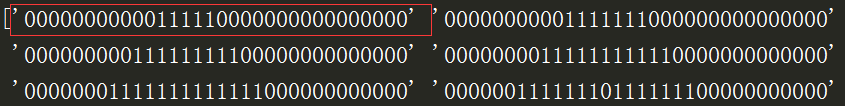解法：拿到这个红框，用list(map(int,红框))   ----》[0,0,0,0,0,0,0,0,0]
2、二维数组的第二行以及往下多行怎么赋值？
解法：用上图列表的下标作为二维数组的行坐标
实践：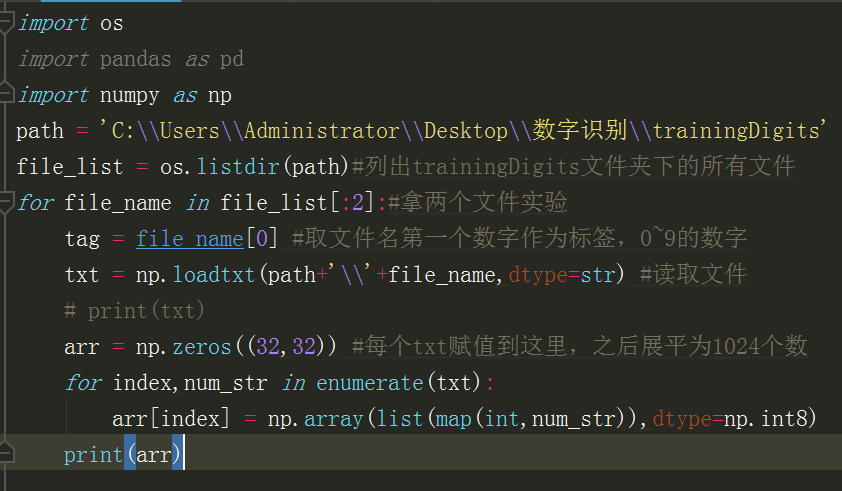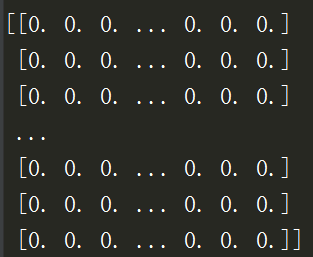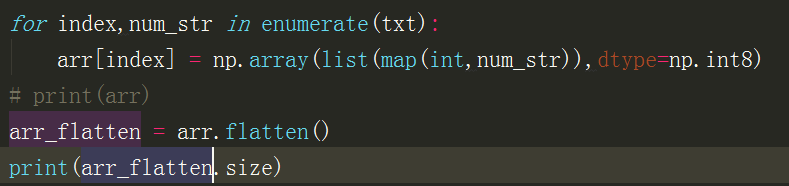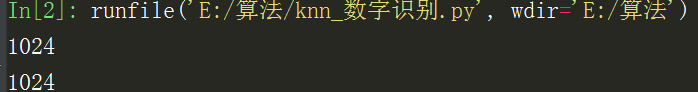（四）将上一步的一行1024个0,1赋值给最终的数组（knn计算的数组）
问题：一个txt文件就是最终数组的一行，这个行的下标怎么定呢？
解决：第一个for就应该取个枚举，所以修改第一个for的代码（还要在第一个for之前定义一个最终数组）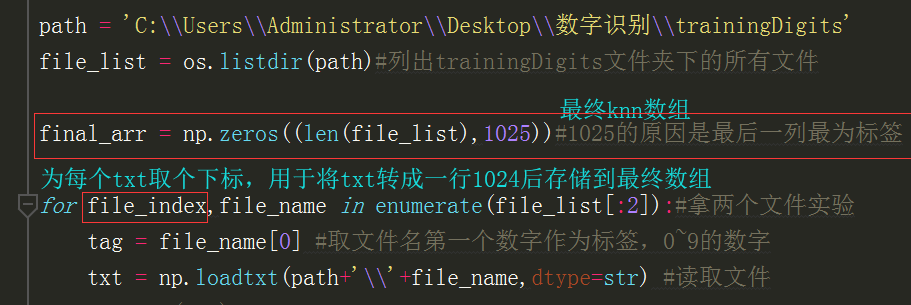加上存储到最终数组的代码后，目前的完整代码如下：（不再拿两个文件，而是拿全部）
import os
import pandas as pd
import numpy as np
file_list = os.listdir(path)#列出trainingDigits文件夹下的所有文件

final_arr = np.zeros((len(file_list),1025))#1025的原因是最后一列最为标签

for file_index,file_name in enumerate(file_list):#拿全部文件
tag = file_name #取文件名第一个数字作为标签，0~9的数字
# print(txt)
arr = np.zeros((32,32)) #每个txt赋值到这里，之后展平为1024个数
for index,num_str in enumerate(txt):
arr[index] = np.array(list(map(int,num_str)),dtype=np.int8)
# print(arr)
arr_flatten = arr.flatten()#展平为一行1024个1,0
# print(arr_flatten.size)
#赋值到最终数组,file_index代表了第几个txt也就是赋值都最终数组的第几行
final_arr[file_index,:-1] = arr_flatten#最后一列没赋值，保留做tag
# 最后一列进行赋值，代表此行数据就是0或1，也就是训练集结果
final_arr[file_index, -1] =tag
（五）将最终数组进行保存为训练集的训练结果（分类器）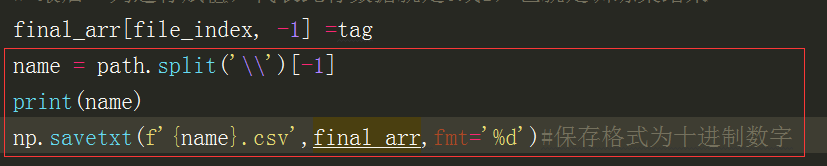（六）训练集完成（分类器）
（训练集制作的完整代码）
import os
import pandas as pd
import numpy as np
file_list = os.listdir(path)#列出trainingDigits文件夹下的所有文件

final_arr = np.zeros((len(file_list),1025))#1025的原因是最后一列最为标签

for file_index,file_name in enumerate(file_list[:2]):#拿两个文件实验
tag = file_name #取文件名第一个数字作为标签，0~9的数字
# print(txt)
arr = np.zeros((32,32)) #每个txt赋值到这里，之后展平为1024个数
for index,num_str in enumerate(txt):
arr[index] = np.array(list(map(int,num_str)),dtype=np.int8)
# print(arr)
arr_flatten = arr.flatten()#二维数组展平为一行
# print(arr_flatten.size)
#赋值到最终数组,file_index代表了第几个txt也就是赋值都最终数组的第几行
final_arr[file_index,:-1] = arr_flatten#最后一列没赋值，保留做tag
# 最后一列进行赋值，代表此行数据就是0或1，也就是训练集结果
final_arr[file_index, -1] =tag
name = path.split('\\')[-1]
print(name)
np.savetxt(f'{name}.csv',final_arr,fmt='%d')#保存格式为十进制数字


（七）测试集制作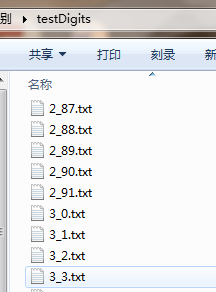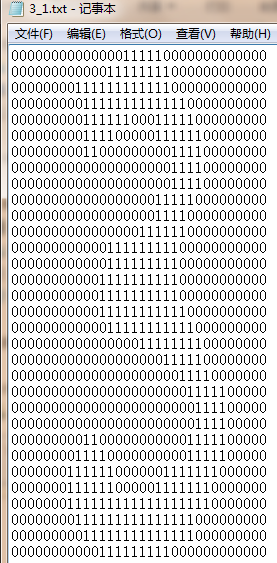32x32
测试集制作与训练集制作流程一样，训练集产生testDigits.csv文件，第1025列也是tag,
现在我们要做的就是：拿testDigits.csv的前1024列对训练集进行测试，测试结果与testDigits.csv的第1025列比对，判断准确率
既然制作流程一致，那就封装个函数：
import os
import pandas as pd
import numpy as np

def data_trans(path):
file_list = os.listdir(path)#列出trainingDigits文件夹下的所有文件
file_list.pop() #trainingDigit最后一个文件有问题就删了
final_arr = np.zeros((len(file_list),1025))#1025的原因是最后一列最为标签

for file_index,file_name in enumerate(file_list):#拿两个文件实验
tag = file_name #取文件名第一个数字作为标签，0~9的数字
# print(txt)
arr = np.zeros((32,32)) #每个txt赋值到这里，之后展平为1024个数

for index,num_str in enumerate(txt):
arr[index] = np.array(list(map(int,num_str)),dtype=np.int8)

# print(arr)
arr_flatten = arr.flatten()
# print(arr_flatten.size)
#赋值到最终数组,file_index代表了第几个txt也就是赋值都最终数组的第几行
final_arr[file_index,:-1] = arr_flatten#最后一列没赋值，保留做tag
# 最后一列进行赋值，代表此行数据就是0或1，也就是训练集结果
final_arr[file_index, -1] =tag
name = path.split('\\')[-1]
print(name)
np.savetxt(f'{name}.csv',final_arr,fmt='%d')#保存格式为十进制数字

if __name__ == '__main__':
data_trans(path1)
data_trans(path2)

以上代码生成两个文件（其实他俩一样，只是一个用来测试，一个用来被测试罢了）：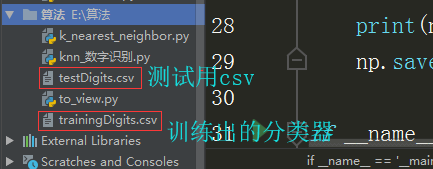（八）进行测试（knn算法）
import numpy as np
import pandas as pd
import matplotlib.pyplot as plt

def knn(final_arr,test_arr,k):
"""
在test_arr中取出每一行用分类器final_arr进行分类给出标签
计算出的标签与test_arr里的标签对比来计算准确率
:param final_arr: 训练出来的分类器
:param test_arr: 测试集
:k: knn中的k，排序后取样本的数量
:return: 准确率
"""
test_arr_rows = test_arr.shape #测试集有多少行
correct_num = 0 # 分类器得到正确tag的个数，用于计算准确率
for i in range(test_arr_rows):
row_to_test = test_arr[i,:-1] #待测试的行
#计算出与分类器每一行的距离（用到数组的广播性质）
distance = np.sqrt(((row_to_test-final_arr[:,:-1])**2).sum(axis=1))
#根据knn算法将距离排序后去前K个做判断标准，
# K个样本中取出标签的众数作为判断结果
#argsort函数返回的是数组值从小到大的索引值
#这个索引值也是final_arr的对应行索引值
distance_sorted_index = distance.argsort()[:k]
#在final_arr中拿到离测试点最近的前k行的tag
k_tags_arr = final_arr[distance_sorted_index,-1]
#取k个tag中的众数，众数函数是在DataFrame中，所以转一下
predict_tag = pd.DataFrame(k_tags_arr).mode()
#mode()返回的是DataFrame，所以取值要predict_tag
print('预测值：',predict_tag)
print('真实值：',test_arr[i,-1])
if predict_tag == test_arr[i,-1]:
correct_num += 1
#因为这个准确度要反映到绘图上，所以不能是字符串，还是在绘图时转成%形式吧
# precision = '%.4f%%'%(correct_num/test_arr.shape)
precision =correct_num/test_arr.shape
# print('准确度为：',precision)
return precision

if __name__ == '__main__':
final_arr = np.loadtxt('testDigits.csv',dtype=int)#[0 0 0 ... 0 0 0]
test_arr = np.loadtxt('trainingDigits.csv',dtype=int)#不加dtype返回的是浮点数[0. 0. 0. ... 0. 0. 0.]
#测试不同k的准确度
x = list(range(5,15))
y = [] #y用来接收不同k返回的准确度，用于绘图
for k in range(5,15):
precision = knn(final_arr,test_arr,k)
y.append(precision)
print(x)#
print(y)#[0.967408173823073]
#绘图
plt.figure()
plt.plot(x,y,marker='*',markersize=12,ha='center')
for a,b in zip(x,y):
plt.text(a,b,'%.3f%%'%(b*100))
plt.xlabel('k')
plt.ylabel('precision')
plt.show()


测试结果图：可以看出k取5,7时准确率很高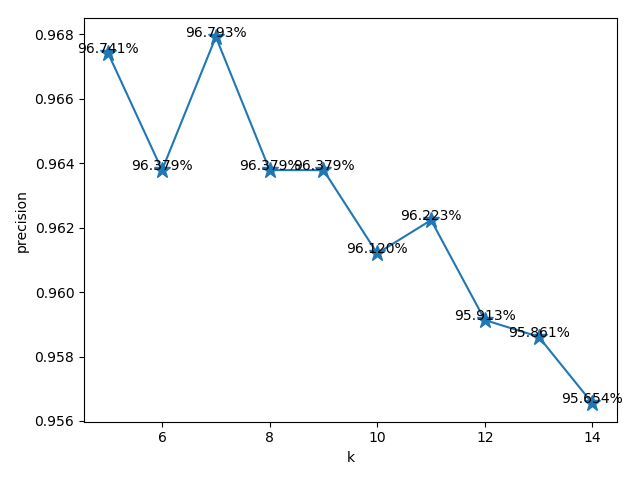展开全文• 识别数字 原理 通过求出测试样例与所有训练样例的距离来判断分类 求出试样例与所有训练样例的距离（小学二年级学的距离公式：距离的平方=(x1-x2)^ 2+(y1-y2)^ 2） 将距离从小到大排序后取前k个 k个中最多是哪个分类...机器学习
• KNN算法使用MNIST数据集、0-1二值图集、自写的数字图片集，做手写数字识别的代码，文件夹分类明确。
• 使用kNN算法对0-9的手写数据集进行识别，包括python代码和数据，数据为txt格式。
• 图片大小为1000*2000,有0-9的10个数字，每5行为一个数字，总共50行，共有5000个手写数字，每个数字块大小为20*20。 为了后续方便处理，我们先写一段小程序把这5000个图截取出来：#include #incl
• 基于KNN数字识别 源代码及数据的github地址： https://github.com/w1449550206/KNN-Handwritten-digit-recognition-based-on-KNN 点此可直达 import numpy as np # 图片后缀为bmp import matplotlib.pyplot as plt...
• 使用opencv库， 基于KNN分类器的ocr(数字识别)，参考了opencv2.0的例子，进行了部分改编，C++的实现，欢迎下载！
• 前言首先需要说明，这里所说的数字识别不是手写数字识别！但凡对机器学习有所了解的人，相信看到数字识别的第一反应就是MNIST。MNIST是可以进行数字识别，但是那是手写数字。我们现在要做的是要识别从九宫格图片中...opencv 机器学习
• 写本文的目的是记录自己学习过或做过的一些东西，深化理解，理清思路，便于回忆。本人处于学习阶段，欢迎指出...数据集使用的是MNIST，使用每个数字1000张，一共10000张作为模板，另外1000张（每个数字100张）作为...
• 可支持手写数字识别，r和python两种语言编写，生成画板鼠标手写输入，也支持上传图片
• 本文章总结了KNN算法字手写数字识别的应用，比较系统的对传统KNN和各种改进的KNN算法进行实现，由于完整的代码量较大（部分代码参考了机器学习实战），不适合全部贴出，但是博主乐于分享，有需要的话可以直接联系我...
• 需要下载MNIST数据集，将路径修改为本地MNIST数据集的地址。需要OpenCV与Tensorflow环境
• 电脑通过串口传给单片机，单片机用KNN算法给出手写数字的预测结果，并且在液晶上显示
• 1.首先要做数字识别的第一步是要收集数据，我们先来看看著名的MNIST数据集 数据集下载网址：http://yann.lecun.com/exdb/mnist/ train-images-idx3-ubyte.gz: training set images (9912422 bytes) train-labels-idx...机器学习
• 前段时间做项目用到一些图像处理的内容，功能不算太难，但却是我第一次使用opencv库、第一次用c++写工程（老c了）、第一次接触KNN算法，还是很有纪念意义的，所以今天在这里记录一下，也算是重新巩固一遍做过的内容...opencv c++ 近邻算法 目标检测
• KNN实现手写数字识别，包含图片数据，已经实现图片数据与文本数据的转换...